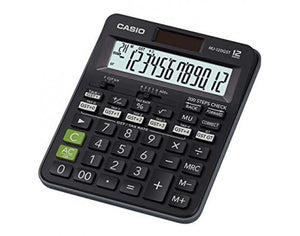# Casio Calculator MJ 120GST

• Rs. 690.00
Tax included.

Casio Calculator MJ 120GST

### GST Calculations

#### Calculation of multiple tax rates

Simple taxable amount, tax amount, and plus-tax amount calculations for five tax rates, and totals for each.#### Calculating price-plus-tax, price-less-tax, and tax grand totals#### Grand totalAutomatic totalization of the results of different calculations.
GT will be operational when last operation is "=" or "%".
* GT will not work when calculator is in "Recall" phase.

#### Calculating the price-plus-tax, price-less-tax, and tax subtotals for each tax rate (recall mode)*1 Price-less-tax subtotal
*2 Tax subtotal
*3 Price-plus-tax subtotal
*4 Price-less-tax grand total calculation
*5 Tax grand total calculation
*6 Price-plus-tax grand total calculation
*7 Pressing any one of the following keys and then the "GST GT" key will perform a GST GT (grand total) operation: "GST+0", "GST+1", "GST+2", "GST+3", "GST+4". In all other cases, pressing the "GST GT" key performs a GT (grand total) operation.

### 200 Steps Check

#### Review

Review up to 200 calculation steps.

Use the [CHECK ← BACK] and [CHECK → FORWARD] keys to scroll through the steps of past calculations. Up to 200 calculation steps can be retained in memory.While reviewing a calculation, you can make changes in values and operators and re-execute to obtain a new result.

* The LCD examples are from an MJ-120GST.

### Localized Number Display

#### Indian Digit Separator* The LCD example is from MJ-120GST.

## Specifications

•TAX- GST+ CalculationAutomatic calculation of price plus tax, price less tax, selling price, and tax amount.

•12 digits

•Regular percentRegular percentage calculations.

•200 steps checkDisplays up to 200 previous calculation steps.

•Solar & BatterySolar powered when light is sufficient, battery powered when light is insufficient.

•Function command signsA symbol (+, -, ×, ÷) on the display indicates the status of operation you are currently performing.

• Rounding selectorF: Floating decimals. CUT: Values are cut off. UP: Values are rounded up. 5/4: Values are rounded off.

• Decimal selector4,3,2,1,0 : Specifies the number of decimal places as 4,3,2,1,0. ADD2: 2 decimal places are assumed for entries and results of all addition and subtraction operations.

•Key rolloverKey operations are stored in a buffer, so nothing is lost even during high-speed input.

•Extra Large displayLarger display makes more data easier to read.

•Plastic keysDesigned and engineered for easy operation.

•Mark-upAll the mark-up capabilities of an adding machine for simplified cost and profit calculations.

## We Also Recommend

### Net Orders Checkout

Item Price Qty Total
Subtotal Rs. 0.00
Shipping
Total